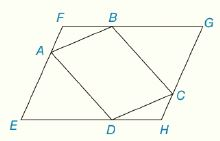Chapter 4.CR, Problem 28CR### Elementary Geometry for College St...

6th Edition
Daniel C. Alexander + 1 other
ISBN: 9781285195698

#### Solutions

Chapter
Section### Elementary Geometry for College St...

6th Edition
Daniel C. Alexander + 1 other
ISBN: 9781285195698
Textbook Problem
13 views

# Review Exercises Given: ∆ F A B ≅ ∆ H C D ∆ E A D ≅ ∆ G C B Prove: A B C D is a ▱To determine

To Prove:

The provided quadrilateral ABCD is a parallelogram.

Explanation

Proof:

Theorem on parallelogram:

If both pairs of opposite sides of a quadrilateral are congruent, then the quadrilateral is a parallelogram.

Description:

It is given that EFGH is a quadrilateral with FABHCD and EADGCB.

Since FABHCD, its sides must be equal in measures. That is, FA=HC, AB=CD and BF=DH.

Since EADGCB, its sides must be equal in measures

### Still sussing out bartleby?

Check out a sample textbook solution.

See a sample solution

#### The Solution to Your Study Problems

Bartleby provides explanations to thousands of textbook problems written by our experts, many with advanced degrees!

Get Started

#### Evaluate the integral. 27. x2+2xdx

Single Variable Calculus: Early Transcendentals

#### By definition the improper integral It is not an improper integral.

Study Guide for Stewart's Single Variable Calculus: Early Transcendentals, 8th

#### The third partial sum of is:

Study Guide for Stewart's Multivariable Calculus, 8th